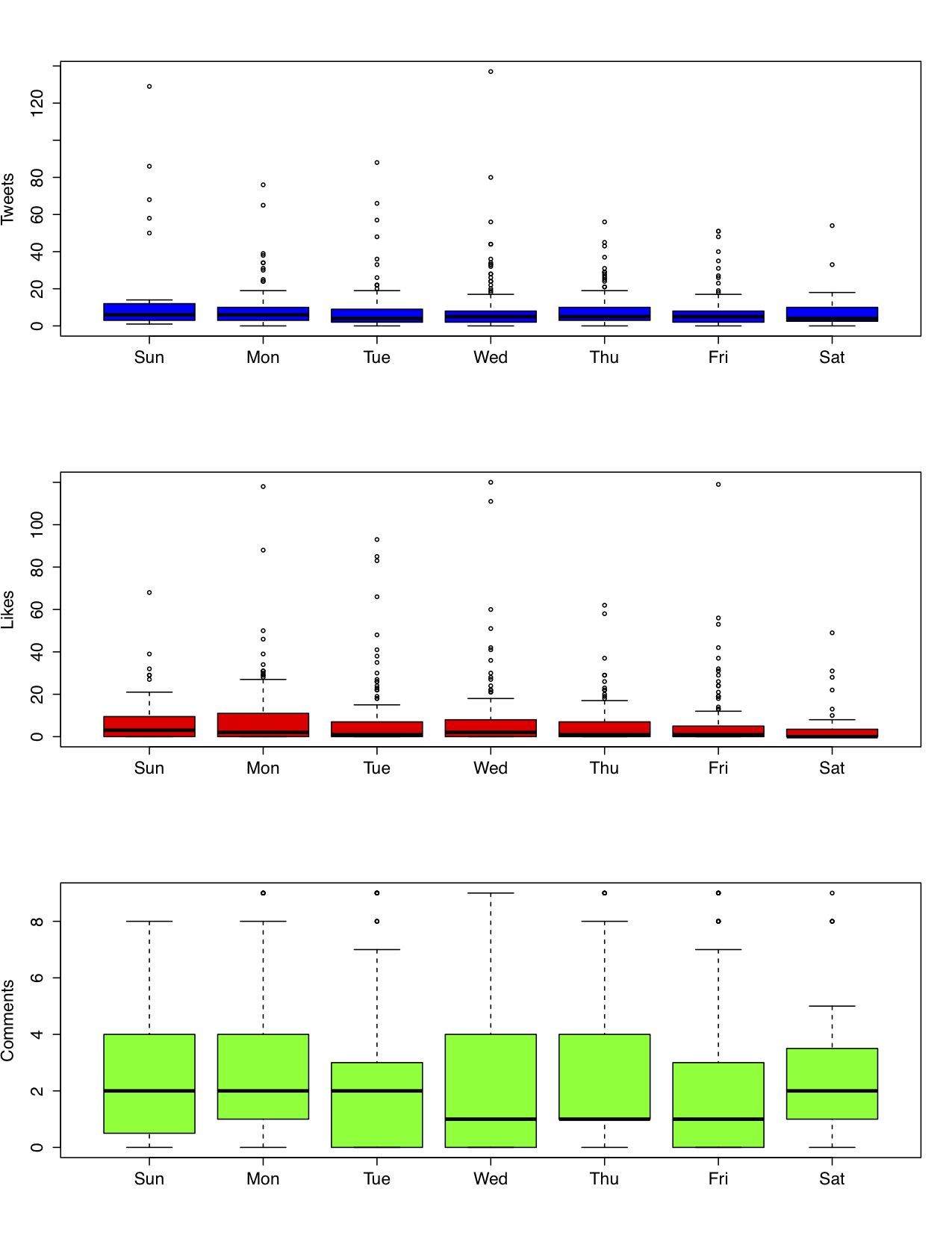This post begins to describe the blog data collected (separately) by Anton Strezhnev and myself. One of the first things I did was to set the date variable in R format so that I could do some exploration.

library(foreign)

monkey1$newdate <- as.Date(monkey1$date, "%m/%d/%Y")

monkey1$weekdaynum <- format(monkey1$newdate, "%w")

day_abbr_list <- c("Sun","Mon","Tue","Wed","Thu","Fri","Sat")

par(mfrow=c(3,1))

boxplot(monkey1$tweets ~ monkey1$weekdaynum, xaxt='n',xlab='',ylab="Tweets",col='blue')
axis(1,labels=day_abbr_list, at=c(1,2,3,4,5,6,7))

boxplot(monkey1$likes ~ monkey1$weekdaynum, xaxt='n',xlab='',ylab="Likes",col='red')
axis(1,labels=day_abbr_list, at=c(1,2,3,4,5,6,7))

boxplot(monkey1$comments ~ monkey1$weekdaynum,xlab='',xaxt='n',ylab="Comments",col='green')
axis(1,labels=day_abbr_list, at=c(1,2,3,4,5,6,7))

[caption id="attachment_717" align="aligncenter" width="640" caption="Monkey Cage Activity by Weekday"][/caption]